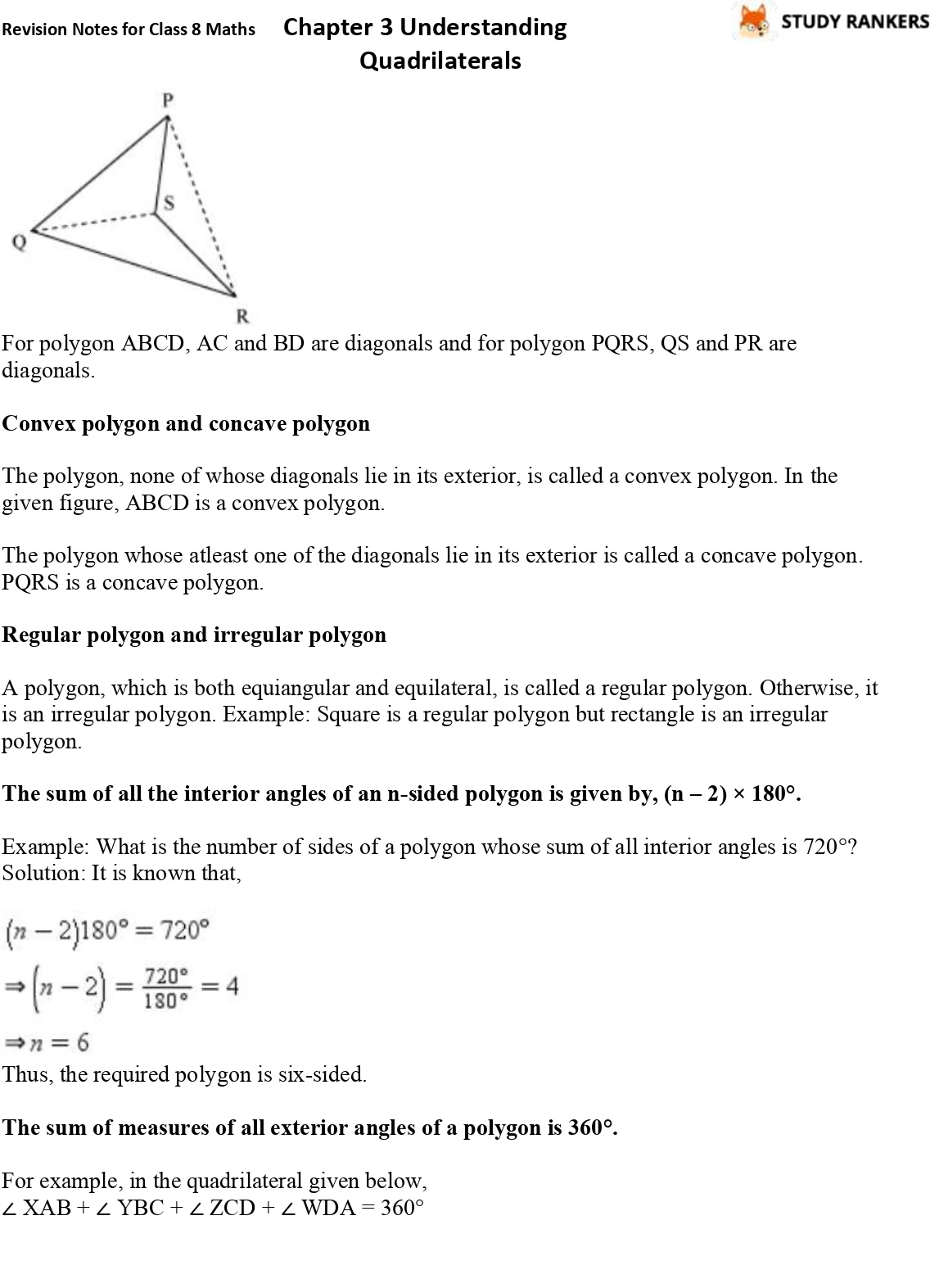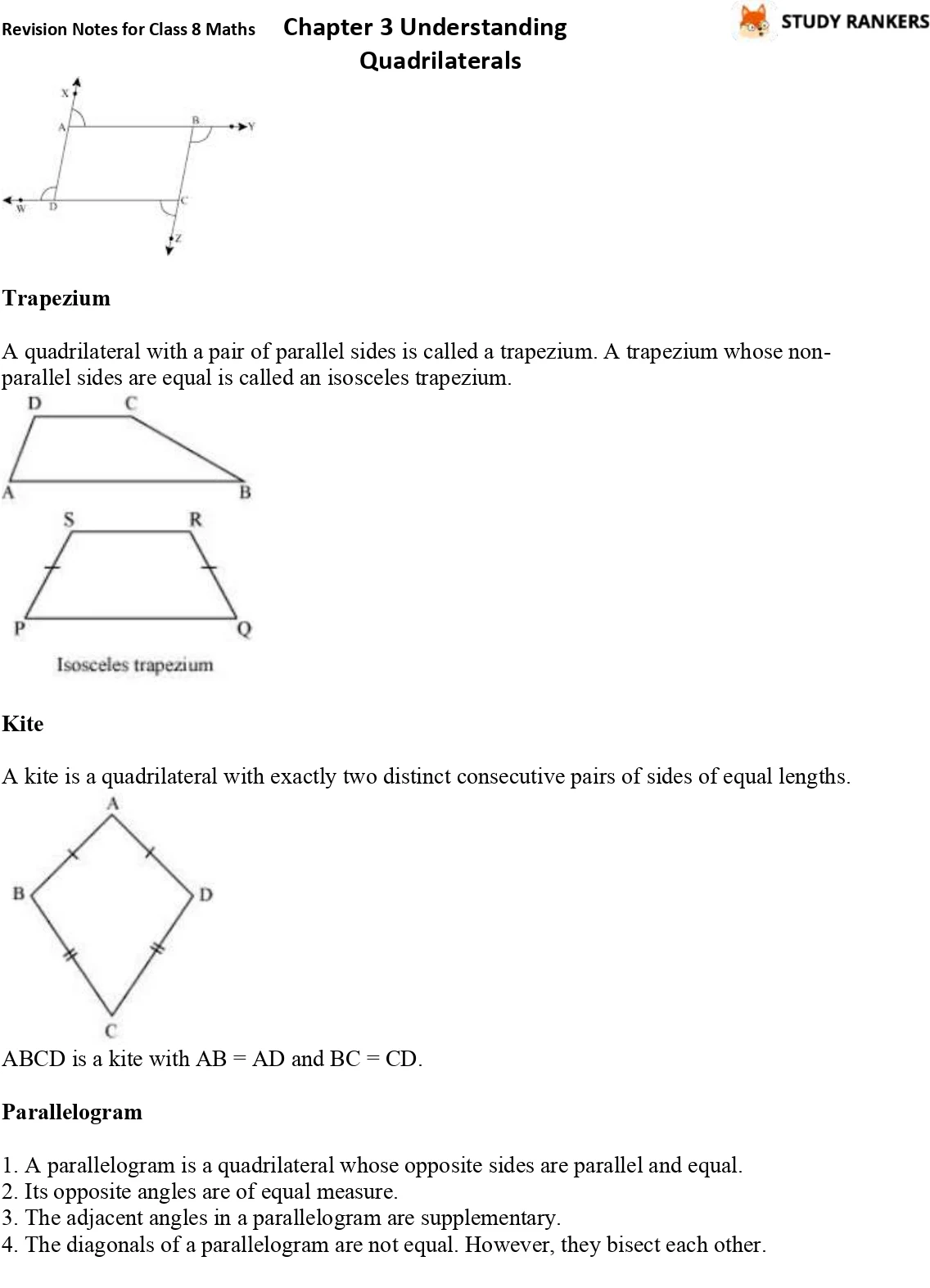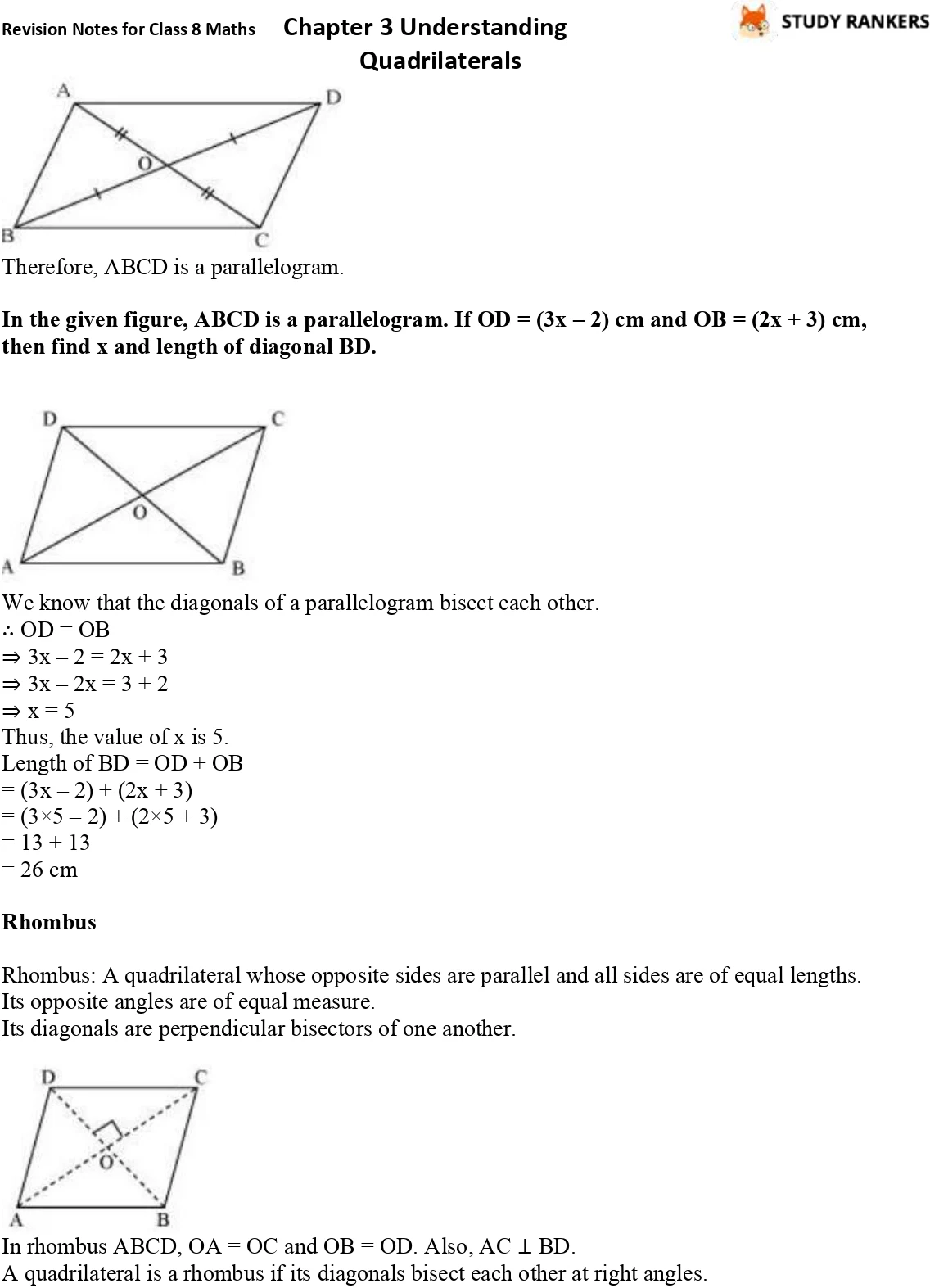## CBSE Revision Notes for Class 8 Chapter 3 Understanding Quadrilaterals

Chapter 3 Understanding Quadrilaterals Revision Notes for Class 8 Maths is provided here which will help you in improving your marks. It will be prove beneficial for every Class 8 students in understanding various topics and revising the concepts. Before examination, you can go through the whole chapter in less time. Class 8 revision notes are prepared by experts faculty of Studyrankers which will teach you the application of theorem.

The topics in the chapter are:
• Polygons
• Diagonal
• Convex polygon and concave polygon
• Regular polygon and irregular polygon
• The sum of all the interior angles of an n-sided polygon is given by, (n – 2) × 180°.
• The sum of measures of all exterior angles of a polygon is 360°.
• Trapezium
• Kite
• Parallelogram
• Opposite sides in a parallelogram are equal.
• Opposite angles in a parallelogram are equal.
• The diagonals of a parallelogram bisect each other.
• Rhombus
• Rectangle
• Square